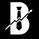Dilihat 447
This study is a proof of concept analysis of situations that can lead to divide with zero or divide by zero conditions. Such situations can lead to mathematical errors, and can increase the potential for false alerts.

One common formula that is prone to this situation is:

pct=(high-close)/(high-low)

This is used to calculate percentage change of a candlestick .

Common outcomes are 0/0, 0/x, x/0, where x is a non zero value.

Visualizing this situation allows for more robust script development and deployment.

There are no user configurations.